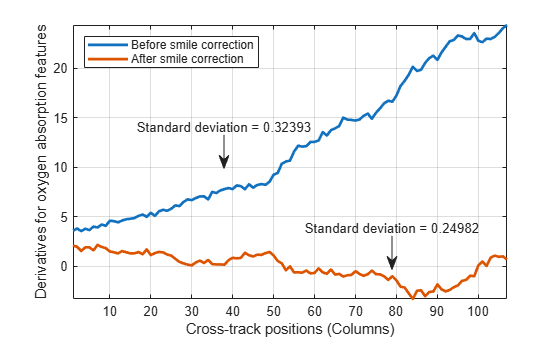smileMetric

Compute spectral smile metrics of hyperspectral data

Description

example

[oxystd,carbonstd,oxyderiv,carbonderiv] = smileMetric(hcube) computes the column mean derivatives, and their standard deviations, for the oxygen and carbon-dioxide absorption features of a hyperspectral data set. You can use these values to detect the spectral smile effect in the hyperspectral data set. For more information, see Smile Indicators.

Note

This function requires the Image Processing Toolbox™ Hyperspectral Imaging Library. You can install the Image Processing Toolbox Hyperspectral Imaging Library from Add-On Explorer. For more information about installing add-ons, see Get and Manage Add-Ons.

Examples

collapse all

Load the hyperspectral data into the workspace.

hcube = hypercube('EO1H0440342002212110PY_cropped.dat');

Compute the column mean derivative values, and their standard deviations, for the oxygen and carbon-dioxide absorption features of the hyperspectral dataset hcube.

[oxystd,carbonstd,oxyderiv,carbonderiv] = smileMetric(hcube);

Perform spectral smile reduction using the maximum noise fraction (MNF) transform-based method.

correctedData = reduceSmile(hcube,'Method','MNF');

Compute the column mean derivative values, and their standard deviations, for the oxygen and carbon-dioxide absorption features of the smile-corrected hyperspectral dataset correctedData.

[noxystd,ncarbonstd,noxyderiv,ncarbonderiv] = smileMetric(correctedData);

Plot the column mean derivative values of the oxygen absorption feature for both the uncorrected hypercube hcube and the smile-corrected hypercube correctedData, and display their standard deviations.

figure
plot(oxyderiv,'LineWidth',2)
hold on
plot(noxyderiv,'LineWidth',2)
hold off
axis tight
grid on
xlabel('Cross-track positions (Columns)')
ylabel('Derivatives for oxygen absorption features')
legend({'Before smile correction','After smile correction'},'Location','northwest');
annotation(gcf,'textarrow',[0.4 0.4],[0.6 0.5],...
'String',['Standard deviation = ' num2str(oxystd)]);
annotation(gcf,'textarrow',[0.7 0.7],[0.3 0.2],...
'String',['Standard deviation = ' num2str(noxystd)]);Plot the column mean derivative values of the carbon-dioxide absorption feature for both the uncorrected hypercube hcube and the smile-corrected hypercube correctedData, and display their standard deviations.

figure
plot(carbonderiv,'LineWidth',2)
hold on
plot(ncarbonderiv,'LineWidth',2)
hold off
axis tight
grid on
xlabel('Cross-track positions (Columns)')
ylabel('Derivatives for carbon-dioxide absorption features')
legend({'Before smile correction','After smile correction'},'Location','southwest');
annotation(gcf,'textarrow',[0.4 0.4],[0.7 0.85],...
'String',['Standard deviation = ' num2str(carbonstd)]);
annotation(gcf,'textarrow',[0.7 0.7],[0.3 0.45],...
'String',['Standard deviation = ' num2str(ncarbonstd)]);Input Arguments

collapse all

Input hyperspectral data, specified as a hypercube object. The DataCube property of the hypercube object stores the hyperspectral data cube. To calculate the column mean of oxygen and carbon-dioxide absorption feature derivatives, the hypercube object must have the full width half maximum (FWHM) values in the Metadata property.

Note

• To compute the column mean of oxygen absorption feature derivatives, the input hyperspectral data must contain data in the visible and near-infrared (VNIR) wavelength range 760 - 785 nm.

• To compute the column mean of carbon-dioxide absorption feature derivatives, the input hyperspectral data must contain data in the short-wave-infrared (SWIR) wavelength range 2010 - 2025 nm.

Output Arguments

collapse all

Column mean derivatives for the oxygen absorption features, returned as a N-element row vector. N is the number of columns in the input hyperspectral data cube. If the input hyperspectral data cube is of type double, then the output vector is of data type double. Otherwise, the data type of the output vector is single.

Column mean derivatives for the carbon-dioxide absorption features, returned as a N-element row vector. N is the number of columns in the input hyperspectral data cube. If the input hyperspectral data cube is of type double, then the output vector is of data type double. Otherwise, the data type of the output vector is single.

Standard deviation of the column mean derivatives for oxygen absorption features, returned as a scalar. You can use this scalar to detect the presence of the spectral smile effect in hyperspectral data. If the value of oxystd is low, then the chances of the data having a smile effect is less in the VNIR range.

Standard deviation of the column mean derivatives for carbon-dioxide absorption features, returned as a scalar. You can use this scalar to detect the presence of the spectral smile effect in hyperspectral data. If the value of carbonstd is low, then the chances of the data having a smile effect is less in the SWIR range.

collapse all

Smile Indicators

The smile effect occurs when hyperspectral data contains significant cross-track curvature with nonlinear disturbances along the spectral dimension. These nonlinear disturbances occur only in data captured using push-broom hyperspectral sensors, such as the Hyperion EO-1 and SEBASS. Based on , you can detect cross-track variation in the oxygen and carbon-dioxide absorption features, due to a possible smile effect, by calculating the first derivatives of the oxygen and carbon-dioxide band images. The first derivative of the adjacent bands ${B}^{\text{'}}$ is calculated using the absorption band image ${B}_{1}$ and the image of the subsequent band ${B}_{2}$, using the equation:

${B}^{\text{'}}=\frac{{B}_{2}-{B}_{1}}{\overline{FWHM}},$

where, $\overline{FWHM}$ is the average FWHM of the two bands ${B}_{1}$ and ${B}_{2}$. This derivative calculation is applicable to both the oxygen and carbon-dioxide absorption band images. The column mean values of the oxygen and carbon-dioxide derivatives can indicate cross-track nonlinearity caused by the spectral smile effect.

• The nonlinear, cross-track column mean of oxygen absorption feature derivative values indicates a spectral smile effect in the VNIR spectrum.

• The nonlinear, cross-track column mean of carbon-dioxide absorption feature derivative values indicates a spectral smile effect in the SWIR spectrum.

 Dadon, Alon, Eyal Ben-Dor, and Arnon Karnieli. “Use of Derivative Calculations and Minimum Noise Fraction Transform for Detecting and Correcting the Spectral Curvature Effect (Smile) in Hyperion Images.” IEEE Transactions on Geoscience and Remote Sensing 48, no. 6 (June 2010): 2603–12. https://doi.org/10.1109/TGRS.2010.2040391.